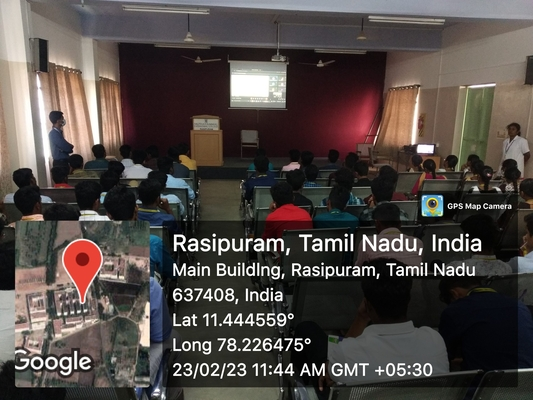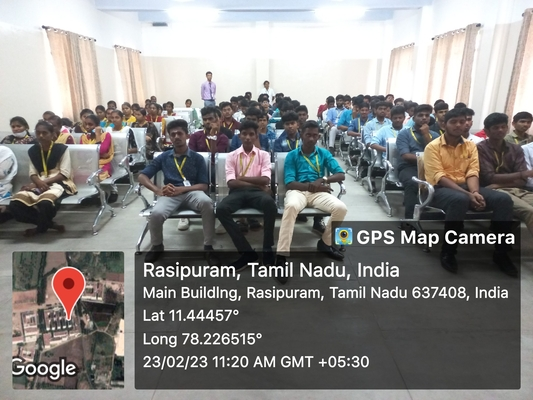# Guest Lecture on Fundamentals on Linear Algebra

• Linear algebra is one of the important branches of mathematics. Linear algebra is basically the study of vectors and linear functions.
• It is a key concept for almost all areas of mathematics. Linear algebra is considered a basic concept in the modern presentation of geometry.
• It is mostly used in Physics and Engineering as it helps to define the basic objects such as planes, lines and rotations of the object.
• It allows us to model many natural phenomena, and also it has a computing efficiency.error: